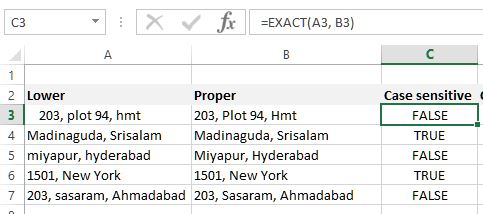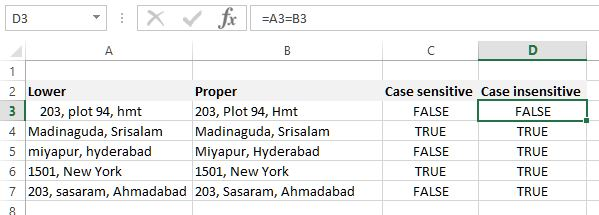# Compare strings in Excel

##### How to compare two strings in Excel using EXACT (Case sensitive) and equal (Case insensitive) operator?

In the previous post, we learnt about how to change string to upper, lower and proper case in Excel. In this post, we shall learn how to compare two strings (case sensitive and case insensitive) in Excel.

### Case Sensitive comparison (EXACT function)

To compare two string (case sensitive comparison), we can use EXACT function. In C3 cell, we have written '=EXACT(A3, B3)' formula that compares A3 and B3 cells and returns FALSE (Notice there is blank space before A3 cell string).

EXACT function returns

1. TRUE - if both strings are exactly same
2. FALSE - if both strings are not exactly same (even blank space matters!)When we copy the same formula to other C cells, we get above result.

### Case Insensitive comparison (= operator)

To compare two strings just for their value, not with their case we can use '=' operator. In D3 cell below we have written '=A3=B3' formula that compares only the string value of both A3 and B3 cells (The blank space matters!).

As A3 has blank space in the start of the string so the result is FALSE, rest all are TRUE as the data are same (Upper case and lower case doesn't matter).When we copy the same formula to other D cells, we get above result.

Views: 2721 | Post Order: 26